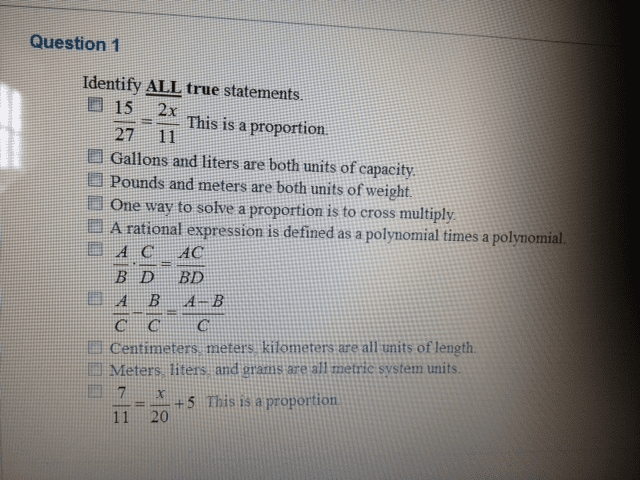1
0
watching
63
views

#Identify ALL true statements 15/27 = 2x/11 This is a proportion. Gallons and liters are both units of capacity. Pounds and meters are both units of weight. One way to solve a proportion is to cross multiply. A rational expression is defined as a polynomial times a polynomial A/B C/D = AC/BD A/C - B/C = A-B/C Centimeters meters, kilometers are all units of length. Meters liters and grams are all metric system units. 7/11 = x/20 + 5. This is a proportion Show transcribed image text Identify ALL true statements 15/27 = 2x/11 This is a proportion. Gallons and liters are both units of capacity. Pounds and meters are both units of weight. One way to solve a proportion is to cross multiply. A rational expression is defined as a polynomial times a polynomial A/B C/D = AC/BD A/C - B/C = A-B/C Centimeters meters, kilometers are all units of length. Meters liters and grams are all metric system units. 7/11 = x/20 + 5. This is a proportion